# Mobious Full Stochastic MTF#### J007RMC

##### Active member
2019 Donor
VIP
Well every evening I read through one note and there I find some gems.. I can be a bit nostalgic at times but then again these gems played a part of where we are today.

# Stochastic Full MTF
# blt
# 7.15.2016

# 07.15.2016 blt v0, initial release
# 07.15.2016 blt v1, added on/off input for bubbles

# Here is a Stochastic Full_MTF indicator coded with a similar look
# as the RSI_Laguerre_Time_MTF uses. On the 1min chart image of DAL,
# I have the Stochastic set to a fast seting of 10,3,1, using higher
# agg period of 2min for the Stochastic.
#
# I used TOS Stochastic Full because with it you can do all three
# flavors (full 10,10,3; fast 10,3,1; slow 10,10,1) just changing
# the inputs
#
# This is an enhanced version with inputs to show bubbles or not.
# If you want to see it in expansion to the right, then you need to
# increase your right expansion to approx 35. The bubbles were
# already moveable at the inputs m and z

declare lower;

input usehigheraggperiod = {default "Current", "Higher"};

# Current uses the current chart agg. So if you are on a 1min chart
# the indicator is based on 1min. If you select higher, then whatever
# agg you enter at the input will be what is used for the RSI. So
# if you are on a 1min chart and higher is set to 2min, then a 2min
# RSI and the entries and targets are 2min based.

input agg = AggregationPeriod.TWO_MIN;
input atrlength = 14;
input atrfactor = 3;

# Variables:
def o;
def h;
def l;
def c;
def error = usehigheraggperiod == usehigheraggperiod."Higher" and GetAggregationPeriod() > agg;
switch (usehigheraggperiod) {
case Current:
o = (open + close) / 2;
h = Max(high, close);
l = Min(low, close);
c = (o + h + l + close) / 4;
case Higher:
if error {
o = Double.NaN;
h = Double.NaN;
l = Double.NaN;
c = Double.NaN;
} else {
o = (open(period = agg) + close(period = agg)) / 2;
h = Max(high(period = agg) , close(period = agg));
l = Min(low(period = agg) , close(period = agg));
c = ((open(period = agg) + close(period = agg)) / 2
+ Max(high(period = agg), close(period = agg))
+ Min(low(period = agg) , close(period = agg))
+ close(period = agg)) / 4;
}
}
AddLabel(error, "Chart Aggregation Period is greater than RSI aggregation period. Need to Change input to a higher agg than current chart aggregation or choose 'Current' at input usehigheraggperiod", Color.WHITE);

#TOS StochasticFull
#used TOS StochasticFull because with it you can do all 3 (full 10,10,3; fast 10,3,1; slow 10,10,1) with it by just changing the inputs for kperiod
input over_bought = 80;
input over_sold = 20;
input KPeriod = 10;
input DPeriod = 10;
input slowing_period = 3;
input averageType = AverageType.SIMPLE;
def priceH = h;
def priceL = l;
def priceC = c;

def lowest_k = Lowest(priceL, KPeriod);
def c1 = priceC - lowest_k;
def c2 = Highest(priceH, KPeriod) - lowest_k;
def FastK = if c2 != 0
then c1 / c2 * 100
else 0;

plot FullK = if isnan(close) then double.nan else MovingAverage(averageType, FastK, slowing_period);
plot FullD = if isnan(close) then double.nan else MovingAverage(averageType, FullK, DPeriod);

plot OverBought = if isnan(close) then double.nan else over_bought;
plot OverSold = if isnan(close) then double.nan else over_sold;

FullK.SetDefaultColor(GetColor(5));
FullD.SetDefaultColor(GetColor(0));
OverBought.SetDefaultColor(GetColor(1));
OverSold.SetDefaultColor(GetColor(1));

def ob = over_bought;
def os = over_sold;
def stou = if FullK crosses above ob or
FullK crosses above os
then 1
else if stou == 1 and !(FullK crosses below ob) and FullK > os
then 1
else 0;
FullK.AssignValueColor(if stou
then Color.GREEN
else Color.RED);

then FullK
else Double.NaN,
OverBought,
Color.GREEN, Color.GREEN);
then OverSold
else Double.NaN,
FullK,
Color.RED, Color.RED);

plot mid = if isnan(close) then double.nan else 50;
plot lineh = if isnan(close) then double.nan else 110;
plot linel = if isnan(close) then double.nan else -10;
lineh.setdefaultColor(color.gray);
linel.setdefaultColor(color.gray);
lineh.hidebubble();
linel.hidebubble();

AddLabel(yes, "STO (" + agg / 60000 + "min): " + Round(FullK, 0),
if FullK > 0
then if FullK > FullK
then Color.DARK_GREEN
else Color.GREEN
else if FullK < FullK
then Color.DARK_RED
else Color.RED);

def atr = Average(TrueRange(h, c, l), atrlength);
def entry = if FullK crosses above OverSold or stou == 0 and FullK crosses above OverBought
then close
else if FullK crosses below OverBought or stou and FullK crosses below OverSold
then close
else entry;
def ebar = if FullK crosses above OverSold or stou == 0 and FullK crosses above OverBought
then BarNumber()
else if FullK crosses below OverBought or stou and FullK crosses below OverSold
then BarNumber()
else Double.NaN;
def target = if FullK crosses above OverSold or stou == 0 and FullK crosses above OverBought
then Round((close + (atrfactor * atr)) / TickSize(), 0) * TickSize()
else if FullK crosses below OverBought or stou and FullK crosses below OverSold
then Round((close - (atrfactor * atr)) / TickSize(), 0) * TickSize()
else target;
def u_d = if FullK crosses above OverSold or stou == 0 and FullK crosses above OverBought
then 1
else if FullK crosses below OverBought or stou and FullK crosses below OverSold
then -1
else u_d;
def goalu = if u_d > 0 and high >= target
then 1
else if goalu == 1 and u_d > 0
then 1
else 0;
def goald = if u_d < 0 and low <= target
then 1
else if goald == 1 and u_d < 0
then 1
else 0;

AddLabel(1, if error then " "
else "T:"+(if u_d > 0 then "Up " else "Dn") + " " + AsText(target) +
(if u_d < 0
then if goald == 0
then " Need " + AsText(Round((target - close), 2))
else " Met "
else "") +
(if u_d > 0
then if goalu == 0
then " Need " + AsText(Round((target - close), 2))
else " Met "
else ""),
if u_d < 0 and !goald or u_d > 0 and !goalu
then Color.WHITE
else Color.GREEN);
input showentrybubble = yes;
input m = 2;
def m1 = m + 1;
if FullK crosses above OverSold or stou and FullK crosses below OverSold
then OverSold
else OverBought,
"Entry: " + AsText(Round(entry, 2)),
if u_d > 0
then Color.GREEN
else Color.RED,
if u_d > 0
then no
else yes);
input showtargetbubble = yes;
if Between(FullK[m1], OverSold, OverBought)
then FullK[m1]
else if u_d[m1] > 0
then 80
else 20,
if error[m1]
then " "
else "T:"+(if u_d[m1] > 0 then "Up " else "Dn ") + " " + AsText(target[m1]) +
(if u_d[m1] < 0 and !goald[m1]
then "\nDiff " + AsText(Round((target[m1] - close[m1]), 2))
else if u_d[m1] > 0 and !goalu[m1]
then "\nDiff " + AsText(Round((target[m1] - close[m1]), 2))
else " Met "),
if u_d[m1] < 0 and !goald[m1] or u_d[m1] > 0 and !goalu[m1]
then Color.WHITE
else Color.GREEN,
if Between(FullK[m1], OverSold, OverBought)
then if u_d[m1] < 0
then no
else yes
else if u_d[m1] < 0
then no
else yes);
#StochasticFull Bubble
input showstochasticbubble = yes;
input z = 30;#Hint z: = number of spaces to move the StochasticFull bubbles sideways
def z1 = z + 1;
mid[z1],
"S",
if stou[z1]
then Color.GREEN
else Color.RED);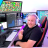How to create a full monitor of many watchlists in ThinkOrSwim? Questions 9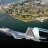Need help with a full time frame continuity scanner? Questions 0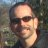MACD and Stochastic with 3/8 ema Questions 6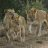RSI Stochastic Strategy Questions 3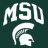Stochastic Scalper for ThinkorSwim Indicators 9